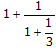Courses

# Unitary Method MCQ 2

## 25 Questions MCQ Test Quantitative Techniques for CLAT | Unitary Method MCQ 2

Description
This mock test of Unitary Method MCQ 2 for Quant helps you for every Quant entrance exam. This contains 25 Multiple Choice Questions for Quant Unitary Method MCQ 2 (mcq) to study with solutions a complete question bank. The solved questions answers in this Unitary Method MCQ 2 quiz give you a good mix of easy questions and tough questions. Quant students definitely take this Unitary Method MCQ 2 exercise for a better result in the exam. You can find other Unitary Method MCQ 2 extra questions, long questions & short questions for Quant on EduRev as well by searching above.
QUESTION: 1

### (16)3/4 is equal to

Solution:

(16)3/4  can be written as ( 2 ) 4 x 3/4

( 2 )  = 8

So option B is the correct answer.

QUESTION: 2

### A number when divided by the sum of 555 and 445 gives two times their difference as quotient and 30 as the remainder. The number is

Solution: Let the number be N
the quotient, in this case, would be = 2(555-445)= 2 x 110 = 220
The Divisor would be = 555+445 = 1000
Remainder = 30
therefore number N would be = Divisor x Quotient + Remainder
which would be = 1000 x 220 + 30 = 220030
QUESTION: 3

### A number divided by 68 gives the quotient 269 and remainder zero. If the same number is divided by 67, the remainder is

Solution:
QUESTION: 4

Given that the number of factors of 12 are 6. The number of factors of 24 must be

Solution:
QUESTION: 5

The difference of two numbers is 3 and the difference of their squares is 39, then the larger number is

Solution:
QUESTION: 6

If (12n + 1) is divisible by 13 then n is

Solution: the answer should be B  I guess because for odd values of N the expression gets divisible by 13.
QUESTION: 7is equal to

Solution:
QUESTION: 8

A man is paid Rs. 20 for each day he works and forfeits Rs. 3 for each day he is idle. At the end of 60 days he gets Rs. 280. Then he was idle for

Solution:
QUESTION: 9

The product of two fractions is 14/15 and their quotient is 35/24, the greater fraction is

Solution:
QUESTION: 10

The sum of squares of two positive numbers is 100 and difference of their squares is 28. The sum of the numbers is

Solution:
QUESTION: 11

Three fourth of two third of a number is 782. What is three fourth of one fourth of the same number

Solution:

Acc to given data,
let the no. be y,
3/4×2/3y = 782
y = 1564
3/4×1/4×1564 = 293.25

QUESTION: 12

A number is 25 more than its two – fifths. Find the number

Solution:
QUESTION: 13

The sum and product of two numbers is 12 and 35 respectively. What is the sum of their reciprocals?

Solution:
QUESTION: 14

A number consists of two digits whose sum is 8. If 18 is subtracted from the number, the digits inter change their places. What is the number.

Solution:
QUESTION: 15

One fourth of a number exceeds its one – seventh by 24. What is the number

Solution:
QUESTION: 16

The sum of the digits of a two digit number is 10. The number formed by reversing the digit is 18 less than the original number. Find the original number.

Solution:
QUESTION: 17

The product of two numbers is 24 times the difference of these numbers. If the sum of these numbers is 14, find the larger number.

Solution:
QUESTION: 18

Find the smallest positive integer which must be subtracted from both the terms of ratio 6 : 7. So that the result gives a ratio, less than 16 : 21

Solution:
QUESTION: 19

The numerator of a certain fraction is increased by 100% and the denominator is increased by 200%. The new fraction thus farmed is 4/21. What is the original fraction?

Solution:
QUESTION: 20

The sum of 18 % of a number and 6% of the same number is 492. What is 12% of that number?

Solution:
QUESTION: 21

The difference between two numbers is 18. If 4 times the second number is less than three times the first number by 18, what is the sum of these two numbers.

Solution:
QUESTION: 22

Five ninth of a number is equal to 2790 what is the number

Solution:
QUESTION: 23

What is the value of two fifth of three-fourth of one – fourth of 26000.

Solution:
QUESTION: 24

If a number is multiplied by three – fourth of itself, value so obtained is 1728. What is the number.

Solution:
QUESTION: 25

What is the value of two – fifth of 60 % of 320?

Solution: